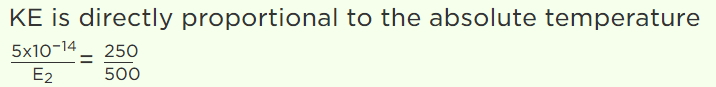## Kinetic Theory Questions and Answers Part-22

1. At a given temperature, the pressure of an ideal gas of density $\rho$ is proportional to
a) $\frac{1}{\rho^{2}}$
b) $\frac{1}{\rho}$
c) $\rho^{2}$
d) $\rho$

Explanation:2. Consider a gas with density $\rho$ and $\bar{C}$ as the root mean square velocity of its molecules contained in a volume. If the system moves as whole with velocity v, then the pressure exerted by the gas is
a) $\frac{1}{3}\rho\bar{C}^{2}$
b) $\frac{1}{3}\rho \left(C+V\right)^{2}$
c) $\frac{1}{3}\rho \left(C-V\right)^{2}$
d) $\frac{1}{3}\rho \left(C^{-2}-V\right)^{2}$

Explanation: $\frac{1}{3}\rho\bar{C}^{2}$

3. If the mean free path of atoms is doubled then the pressure of gas will become
a) P/4
b) P/2
c) P/8
d) P

Explanation: If the mean free path of atoms is doubled then the pressure of gas will become P/2

4. Relationship between P,V, and E for a gas is
a) $P=\frac{3}{2}EV$
b) $V=\frac{2}{3}EP$
c) $PV=\frac{3}{2}E$
d) $PV=\frac{2}{3}E$

Explanation: Relationship between P,V, and E for a gas is $PV=\frac{2}{3}E$

5. The value of universal gas constant is 8.3 J/mole/K, the mean kinetic energy of 32 gm of oxygen at – 73°C will be
a) 480 J
b) 4980 J
c) 2490 J
d) The information is incomplete

Explanation:6. For a diatomic gas change in internal energy for unit change in temperature for constant pressure and constant volume is $U_{1}$  and $U_{2}$  respectively. $U_{1}:U_{2}$  is
a) 5 : 3
b) 3 : 5
c) 1 : 1
d) 5 : 7

Explanation: $U_{1}:U_{2}$  is 1 : 1

7. If number of molecules of $H_{2}$ are double than that of $O_{2}$ , then ratio of kinetic energy of hydrogen and that of oxygen at 300 K is
a) 1 : 1
b) 1 : 2
c) 2 : 1
d) 1 : 16

Explanation: If number of molecules of $H_{2}$ are double than that of $O_{2}$ , then ratio of kinetic energy of hydrogen and that of oxygen at 300 K is 1 : 1

8. The average kinetic energy of a gas at – 23°C and 75 cm pressure is $5 \times10^{-14}erg$   for $H_{2}$ . The mean kinetic energy of the $O_{2}$ at 227°C and 150 cm pressure will be
a) $80 \times10^{-14}erg$
b) $20 \times10^{-14}erg$
c) $40 \times10^{-14}erg$
d) $10 \times10^{-14}erg$

Explanation:9. The ratio of mean kinetic energy of hydrogen and oxygen at a given temperature is
a) 1 : 16
b) 1 : 8
c) 1 : 4
d) 1 : 1